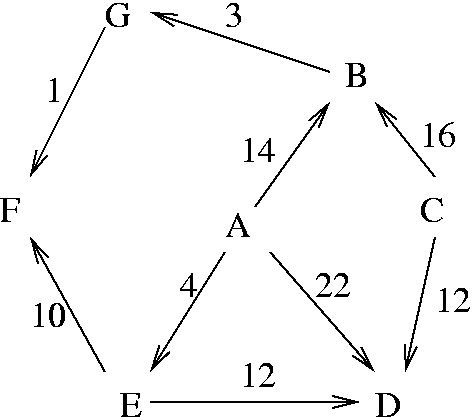# Dijkstra’s Algorithm Example

Dijkstra’s algorithm is a graph algorithm which was invented by the Dutch computer scientist Edsger Dijkstra in 1959. The algorithm finds the shortest ways from one of the graph nodes to all the others.

The algorithm exists in many variants; Dijkstra’s original variant found the shortest path between two nodes but a more common variant fixes a single node as the “source” node and finds the shortest paths from the source to all other nodes in the graph, producing a shortest-path tree (wikipedia.org, 2002).

The algorithm works as follows:

Choose the source node

1. Choose the source node.
2. Define set Z of nodes, and initialise it as an empty set. During the progress of the algorithm, the set Z will contain the nodes to which the shortest path was found.
3. Mark the source node as 0, insert it into Z.
4. Consider each node not in Z connected by an edge from the inserted node. Mark the node outside Z with the mark of the inserted node + the weight of the edge.
• If the node outside Z was already marked, its new mark will be min(mark of the inserted node + the length of edge, old mark)
5. Choose a node outside of Z with the smallest mark, then add it to Z.
6. 6. Repeat from step 4, until the destination node is in Z or there are no marked vertices not in Z.

Example:1. A has mark 0. Z = {A}.
2. A has mark 0, B has 14, D has 22, E has 4. Z={A,E}.
3. A is 0, B has mark 14, D has been marked 16, F has 14. We inserted F. Z={A,E,F}.
4. No change to marks. Z={A,E,F,B}.
5. Labels as before, and G has 17. Z={A,E,F,B,D}.
6. No change to marks. Z={A,E,F,B,D,G}.
7. Stop, as there are no marked nodes not in Z.

Java implementation:

```import java.util.*;

public class Dijkstra {

ArrayList<Node> searchPath(Node[] node, Edge[] edge, Node destination) {
int[][] Weight = createWeight(node, edge);
int[] B = new int[node.length];
Node[] L = new Node[node.length];
ArrayList<Node> K = new ArrayList<Node>();

for (int i = 0; i<node.length; i++) {
K.append(node[i]);
B[i] = Weight[i];
if (B[i] != Integer.MAX_VALUE) {
L[i] = node;
}
}

for (int i = 0; i<node.length - 1; i++) {

int l = Integer.MAX_VALUE;
Node n = node;
for (Node j : K) {
if (B[j.name] < l) {
n = j;
l = B[j.name];
}
}
K.remove(n);

for (int j = 0; j<node.length - 1; j++) {
if (B[n.name] != Integer.MAX_VALUE && Weight[n.name][j] != Integer.MAX_VALUE && B[n.name] + Weight[n.name][j] < B[j]) {

B[j] = B[n.name] + Weight[n.name][j];
L[j] = n;
}
}
}

K.clear();
int loc = destination.name;
K.append(destination);

while (L[loc] != node) {
if (L[loc] == null) {

return null;
}
K.append(0, L[loc]);
loc = L[loc].name;
}
K.append(0, node);
return K;
}

private int[][] createWeight(Node[] node, Edge[] edge) {
int[][] Weight = new int[node.length][node.length];
for (int i = 0; i<node.length; i++) {
Arrays.fill(Weight[i], Integer.MAX_VALUE);
}
for (Edge e : edge) {
Weight[e.from.name][e.to.name] = e.weight;
}
return Weight;
}
}
```

Most students prefer to use our Dijkstra’s algorithm example to deal with their own assignments. But even after reading our sample several times, you may not be able to deal with your homework on your own. Students often realize they need to get professional assignment help in order to succeed in their studies. You may begin with getting help from AssignmentShark.com.

Our Dijkstra’s algorithm example was developed by an expert. If you need the same homework to be done, you just need to place an order on our site with your requirements and the deadline. Let’s say you have a helper who can solve all your problems with your assignment. We have reasonable prices, so any student can afford using our service.

You can spend your time as you want while your expert is completing your order. You don’t need to wait for a long time, as our writers work fast and your order will be completed even before the deadline. We work 24/7, so it is rather convenient for you to use our service anytime you need. Our service is safe – all your personal information will be secure and never passed to third parties. Our expert knows exactly how your assignment should be done. Leave your homework problems to us and we will solve them as soon as possible.